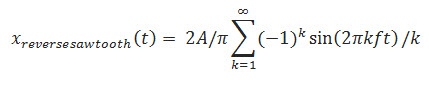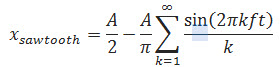# Sawtooth Wave Generator and its Working Principle

A waveform is a shape that represents changes in amplitude with respect to time. A periodic waveform includes a sine wave, square wave, triangular wave, sawtooth wave. On the x-axis, it indicates the time and on the y-axis it indicates amplitude. Many people frequently get confused between the triangular wave and a sawtooth wave. The sawtooth wave generator is one kind of linear, nonsinusoidal waveform, and the shape of this waveform is a triangular shape in which the fall time and rise time are different. The sawtooth waveform can also be named an asymmetric triangular wave.

## Sawtooth Wave Generator

A linear, non-sinusoidal, triangular shape waveform represents a sawtooth waveform in which fall time and rise time are different. A linear, non-sinusoidal, triangular shape waveform represents a pure triangular waveform in which fall time and rise times are equal. The Sawtooth Wave Generator is also known as an asymmetric triangular waveform. The graphical representation of a sawtooth waveform is given below:

The applications of a sawtooth waveform are in frequency/tone generation, sampling, thyristor switching, modulation, etc.

A non-sinusoidal waveform is nothing but a sawtooth waveform. Because its teeth look like a saw, it is named as a sawtooth waveform. In an inverse (or reverse) sawtooth waveform the wave suddenly ramps downwards and then rises sharply.

The infinite Fourier series isA conventional sawtooth can be constructed usingWhere A is the amplitude

By using a fast Fourier transform, this summation can be calculated more efficiently. In the time domain, the waveform is digitally created by using the non-band limited form. Sampling the infinite harmonics results in the tone that contains aliasing distortion.

### Working Principle of a Sawtooth Wave Generator using 555

A sawtooth wave generator can be constructed using a transistor and a simple 555 timer IC, as shown in the below circuit diagram. It consists of a transistor, a capacitor, a Zener diode, resistors from a constant current source that are used to charge the capacitor. Initially, let us assume that the capacitor is fully discharged. The voltage across the capacitor is zero and the 555’s output is high because of the internal comparators connected to the pin 2.

The capacitor starts charging to supply voltage because the internal transistor of 555 shorting the capacitor to ground and it opens. During charging, the 555 output goes low if the voltage increases above 2/3rd of the supply voltage. During discharging, the 555 output goes high if the voltage across C decreases below 1/3rd supply voltage. Hence the capacitor charges and discharges between 2/3rd and 1/3rd of the supply voltage. But the disadvantage is that it requires a bipolar power supply. The frequency is given by

F = (Vcc-2.7) / (R*C*Vpp)

Where,

Vpp- Peak to peak output voltage

Vcc- Supply voltage

To get the required frequency value, select the proper values for Vcc, Vpp, R, and C

### Sawtooth Wave Generator using OP-AMP

A sawtooth waveform is used in pulse width modulation circuits and time-base generators. A potentiometer is used when the wiper moves toward negative voltage(-V); then the rise time becomes more than the fall time. When the wiper moves towards positive voltage(+V), then the rise time becomes less than the fall time.

When the comparator output goes negative saturation, a negative voltage is added to the inverting terminal, thereby the wiper moves to a negative supply. This causes a decrease in the potential difference across R1 and hence current through the capacitor and resistor decreases.

Then the slope decreases and rise time also decrease. When the comparator output is under positive saturation, the potential difference across the R1 increases and current through the capacitor resistor also increases. This is due to the presence of a negative voltage at the inverting terminal. Then the slope increases and fall time decreases. And the output is obtained as a sawtooth waveform.

For wiring the circuit, the following are the components:

• Op-amp IC- 741c
• R-47K
• R1- 1K
• R2- 180Ω

### What is a Sine Wave?

A mathematical curve that describes a smooth repetitive oscillation is said to be a sine wave or sinusoid wave. Often it occurs in pure and in signal processing as well as physics, chemistry, applied mathematics and in many other fields. It is the function of time (t). When added to any other sine wave with the same frequency, phase and magnitude, the sine wave retain its wave shape. It is known to be a periodic waveform that has this type of property. Such importance leads to its usage in Fourier analysis.

Y(x, t) = A sin (kx-ωt+Φ) +D

A is the amplitude
ω = 2πf, is the angular frequency
f is the frequency and it is defined as the number of oscillations per second.
Φ is the phase angle
D is non-zero center amplitude

#### What is Cosine Wave?

The shape of the cosine wave is identical to that of the sine wave except that the cosine wave exactly occurs ¼ cycles earlier than the corresponding sine wave. Sine wave and cosine wave have the same frequency, but the cosine wave leads sine wave by 90˚.

Y = cos x

#### Applications

• The sawtooth waveform is the most common waveform used to create sounds with subtractive virtual and analog music synthesizers. Therefore, it is used in music.
• The sawtooth is the form of horizontal and vertical deflection signals that are used to generate a raster on monitor screens or CRT based television.
• The magnetic field suddenly gets collapsed on the wave’s cliff, which causes the resting position of its electron beam as quickly as possible.
• The magnetic field produced by the deflection yoke drags the electron beam on the wave’s ramp, creating a scan line.
• With much lower frequency, the vertical deflection operates in a similar way as the horizontal deflection system.
• The stability of electronic components improves, and hence, there is no need to adjust the picture’s horizontal or vertical linearity.
• The positive voltage causes a deflection in one direction; the negative voltage causes the deflection in other, and the center-mounted deflection uses the screen area to depict a trace.
• The ramp portion must appear as a straight line; otherwise, it indicates the magnetic field, which is produced by the deflection yoke, as not linear,. This results in non-linearity and the television image is squished. Hence, on that side of the picture, the electron beam spends more time.

This is all about a Sawtooth wave generator and its working principle. We believe that the information given in this article is helpful for you for a better understanding of this project. Furthermore, for any queries regarding this article or any help in implementing the electrical and electronics projects, you can feel free to approach us by connecting in the comment section below. Here is a question for you, what is the working principle of the sawtooth wave generator?

Photo Credits: